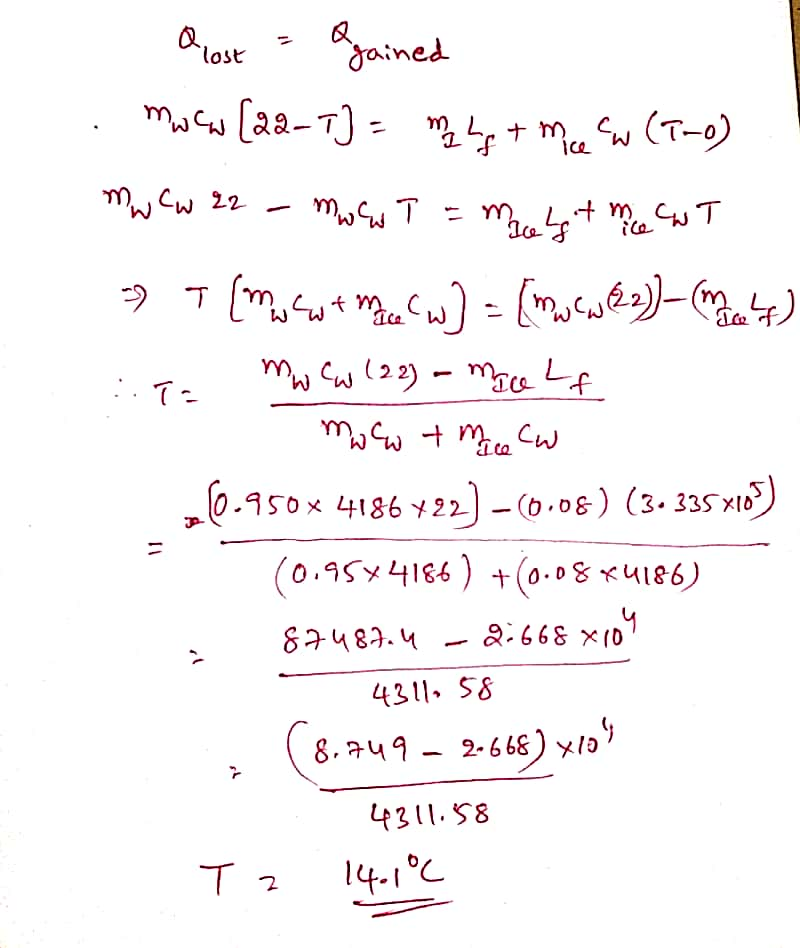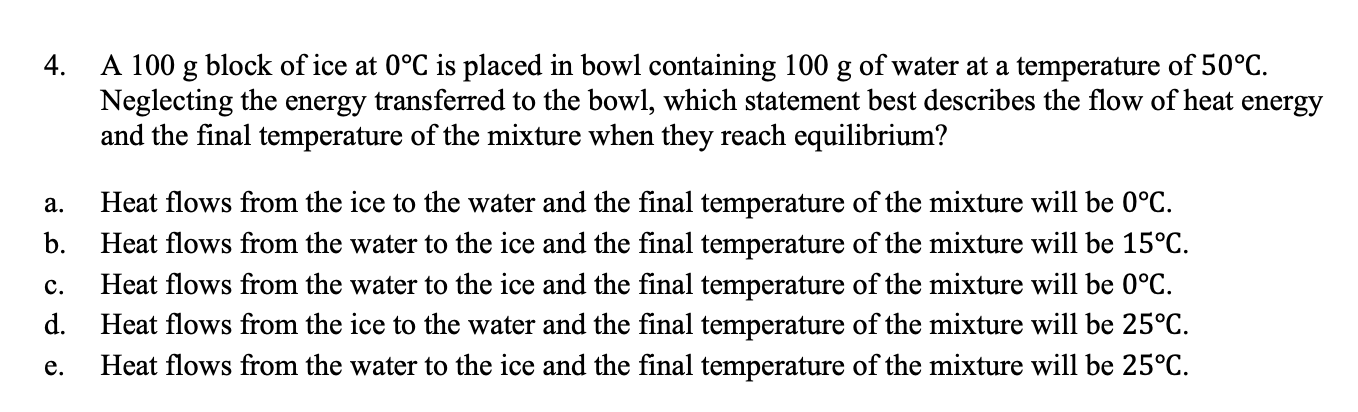Question

A(n) 80-g ice cube at 0°C is placed in 950 g of water at 22°C. What is the final temperature of the mixture?#### Earn Coins

Coins can be redeemed for fabulous gifts.

Similar Homework Help Questions
• ### A(n) 80-g ice cube at 0°C is placed in 750 g of water at 22°C. What...

A(n) 80-g ice cube at 0°C is placed in 750 g of water at 22°C. What is the final temperature of the mixture? _________C

• ### A(n) 90-g ice cube at 0°C is placed in 680 g of water at 26°C. What...

A(n) 90-g ice cube at 0°C is placed in 680 g of water at 26°C. What is the final temperature of the mixture?

• ### 4) A(n) 70-g ice cube at 0°C is placed in 670 g of water at 30°C....

4) A(n) 70-g ice cube at 0°C is placed in 670 g of water at 30°C. What is the final temperature of the mixture? °C

• ### A 74-g ice cube at 0°C is placed in 751 g of water at 28°C. What...

A 74-g ice cube at 0°C is placed in 751 g of water at 28°C. What is the final temperature of the mixture?

• ### A 74-g ice cube at 0°C is placed in 841 g of water at 30°C. What...

A 74-g ice cube at 0°C is placed in 841 g of water at 30°C. What is the final temperature of the mixture?

• ### A 81-g ice cube at 0°C is placed in 930 g of water at 30°C. What...

A 81-g ice cube at 0°C is placed in 930 g of water at 30°C. What is the final temperature of the mixture?

• ### A 85-g ice cube at 0°C is placed in 670 g of water at 26°C. What is the final temperature of...

A85-g ice cube at 0°C is placed in670g of water at26°C. What is the finaltemperature of the mixture?°C

• ### A 85-g ice cube at 0°C is placed in 670 g of water at 26°C. What is the final temperature of...A85-g ice cube at 0°C is placed in670g of water at26°C. What is the finaltemperature of the mixture?Your response differs from the correct answer by more than 10%. Double check your calculations.°C

• ### A 82 g cube of ice at 0°C is dropped into 1.0 kg of water that...

A 82 g cube of ice at 0°C is dropped into 1.0 kg of water that was originally at 80°C. What is the final temperature of the water after the ice has melted? The specific heat of ice is 2090 J/kg°C, and the latent heat of fusion of ice is 3.33x105 J/kg.

• ### 4. A 100 g block of ice at 0°C is placed in bowl containing 100 g...4. A 100 g block of ice at 0°C is placed in bowl containing 100 g of water at a temperature of 50°C. Neglecting the energy transferred to the bowl, which statement best describes the flow of heat energy and the final temperature of the mixture when they reach equilibrium? a. b. c. d. e. Heat flows from the ice to the water and the final temperature of the mixture will be 0°C. Heat flows from the water to the...